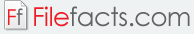### Engineering Equation Solver

#### F-Chart Software, fchart.com (Shareware)

Engineering Equation Solver can numerically solve coupled non-linear algebraic and differential equations for users working in the field of mechanical engineering. It can solve integral and differential equations, as well as convert units, check unit consistency, do optimizations, provide uncertainty analysis and generate publication-quality plots. The program performs iterative solving on thermodynamics and heat-transfer problems inputted by the user by acquiring thermodynamic properties with built-in properties.

Engineering Equation Solver has parametric table that allow the user to view and compare multiple variables and the parametric tables that can be used in generating plots. Optimization tools are included to minimize or maximize variables by tweaking multiple variable equations. The user can store and view information codes in generated look-up tables. Information codes allow the user to enter equations and achieve a solution containing “if then” statements and combined with “if then else” statements. Codes can be written with functions by the user together with procedure functions with multiple outputs. A heat-transfer library is included for convections, conductions, and radiation. It can solve multiple non-linear equations simultaneously, and has single and multi-variable optimization capabilities.

Other features are:

• Automatic unit consistency checks and unit conversions.
• Thermodynamic and transport functions for hundreds of fluids.
• Regression capability and uncertainty analysis.
• Graphical input and output with diagram window.
• 2D and 3D contour-plotting with automatic updates.